# RS Aggarwal Solutions for Class 8 Maths Chapter 1 - Rational Numbers Exercise 1A

Students can refer and download RS Aggarwal Solutions for Class 8 Maths Chapter 1- Exercise 1A, Rational Numbers from the links provided below. Our experts have solved the RS Aggarwal Solutions to ensure that the students are thorough with the basic concepts of the solutions.

In Exercise 1A of RS Aggarwal Class 8 Maths, we shall study the properties of rational numbers using various operations. Firstly what are rational numbers? The numbers of the form a/b, where a and b are integers and b≠0, are called rational numbers.

## Download PDF of RS Aggarwal Solutions for Class 8 Maths Chapter 1- Rational Numbers Exercise 1A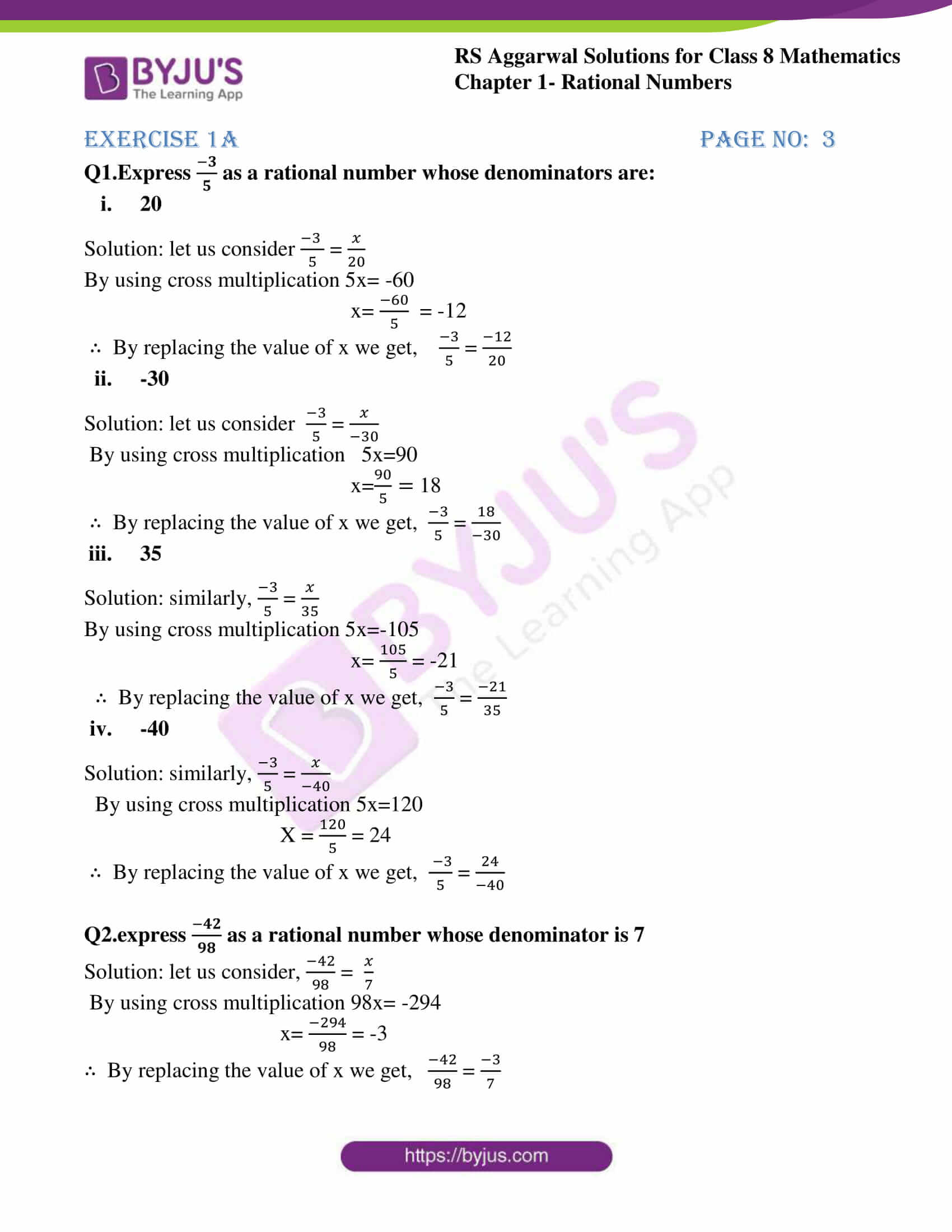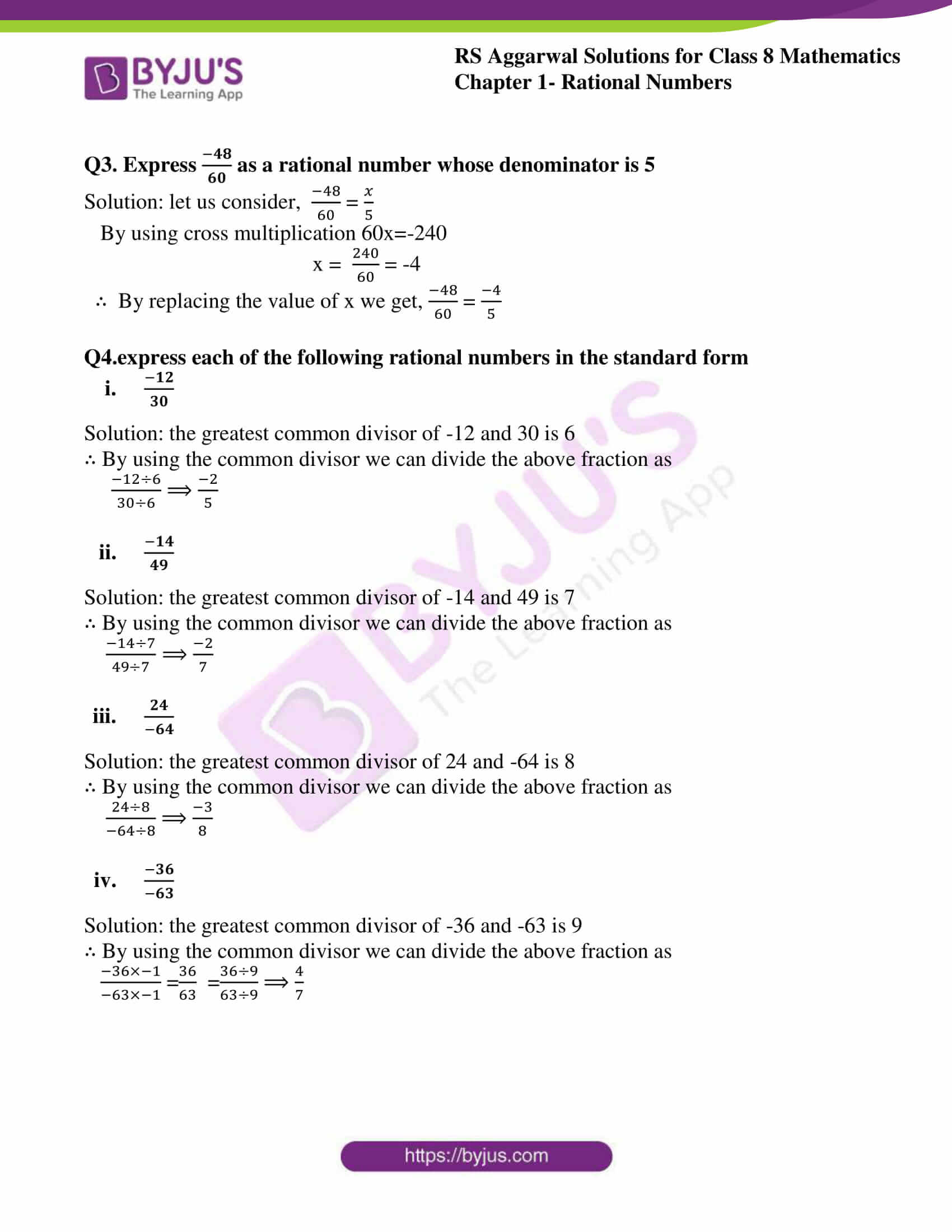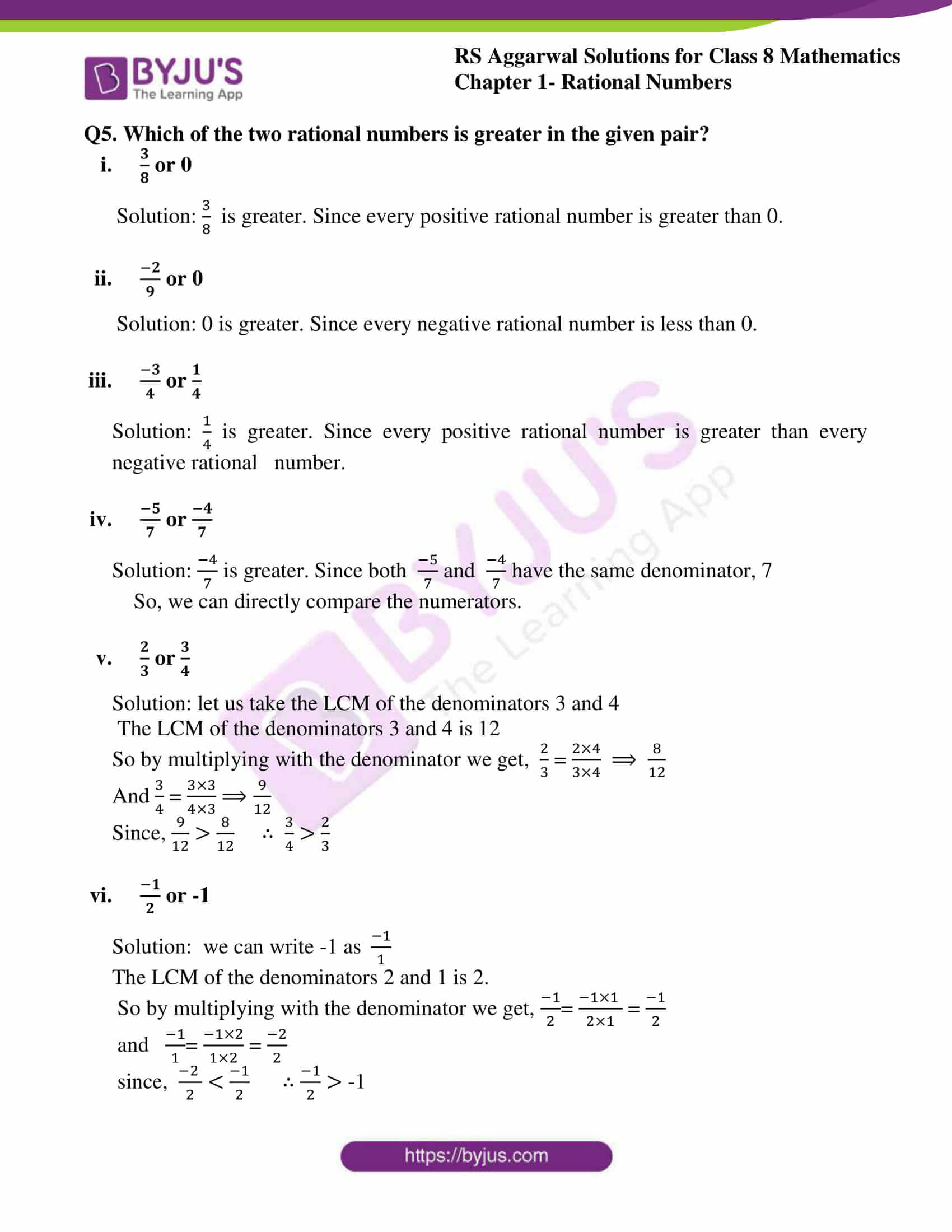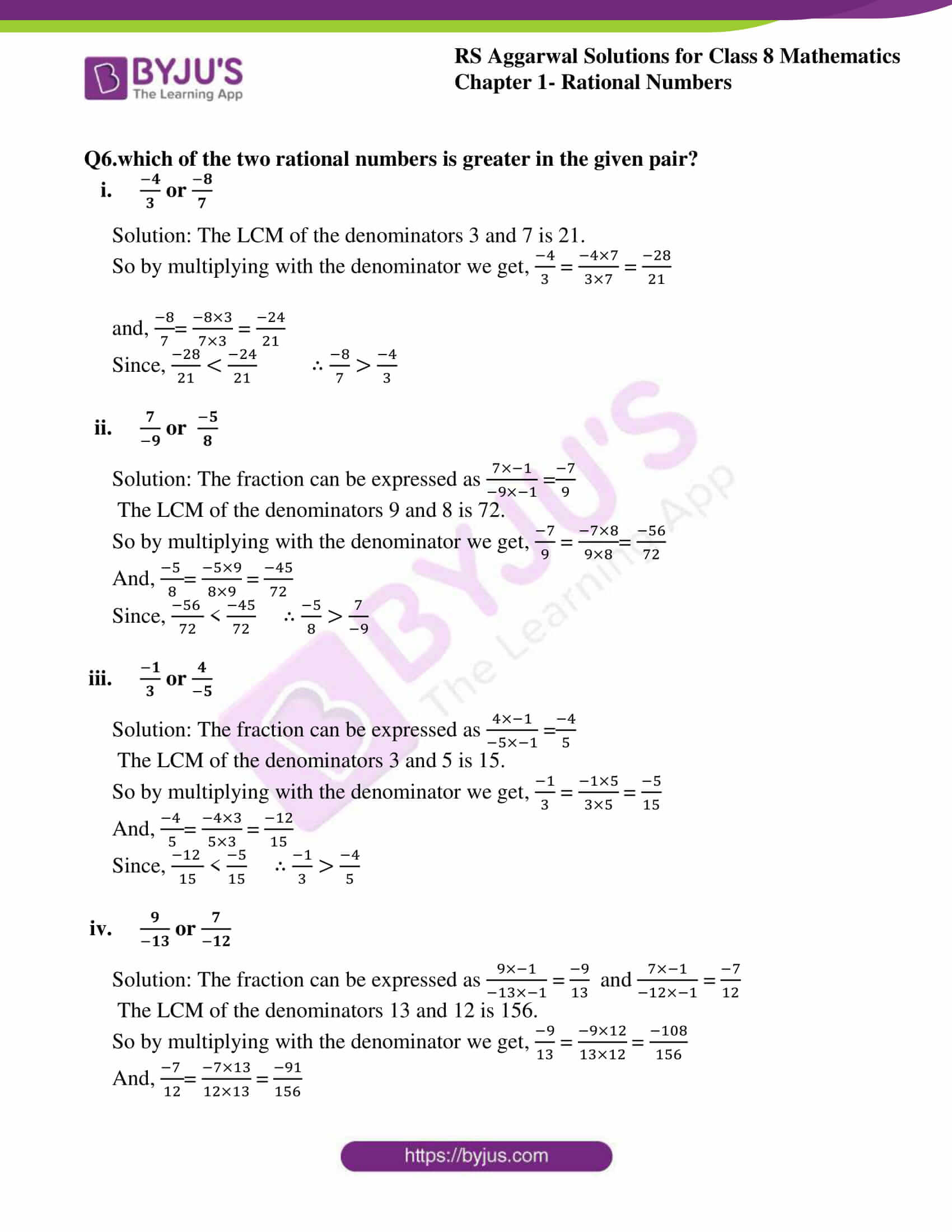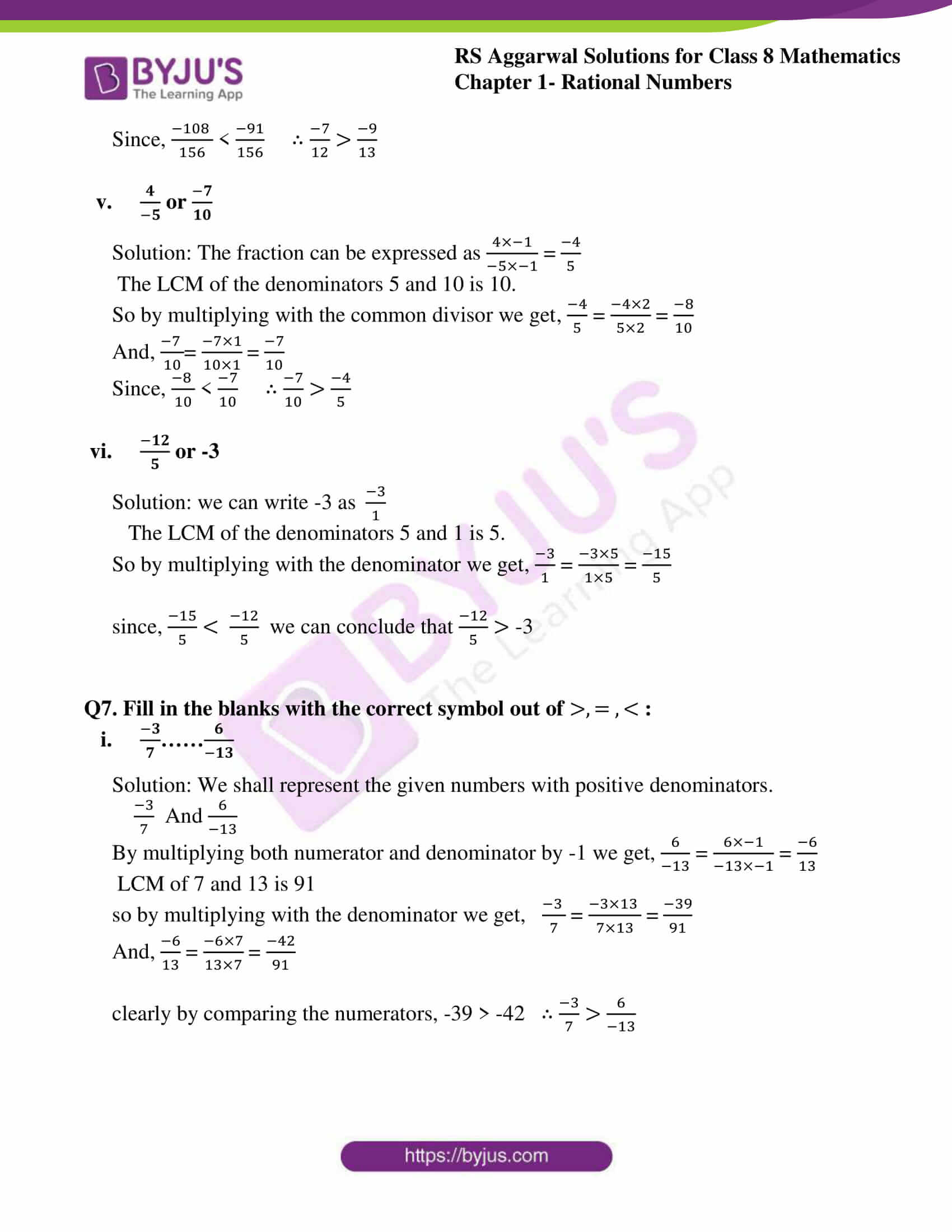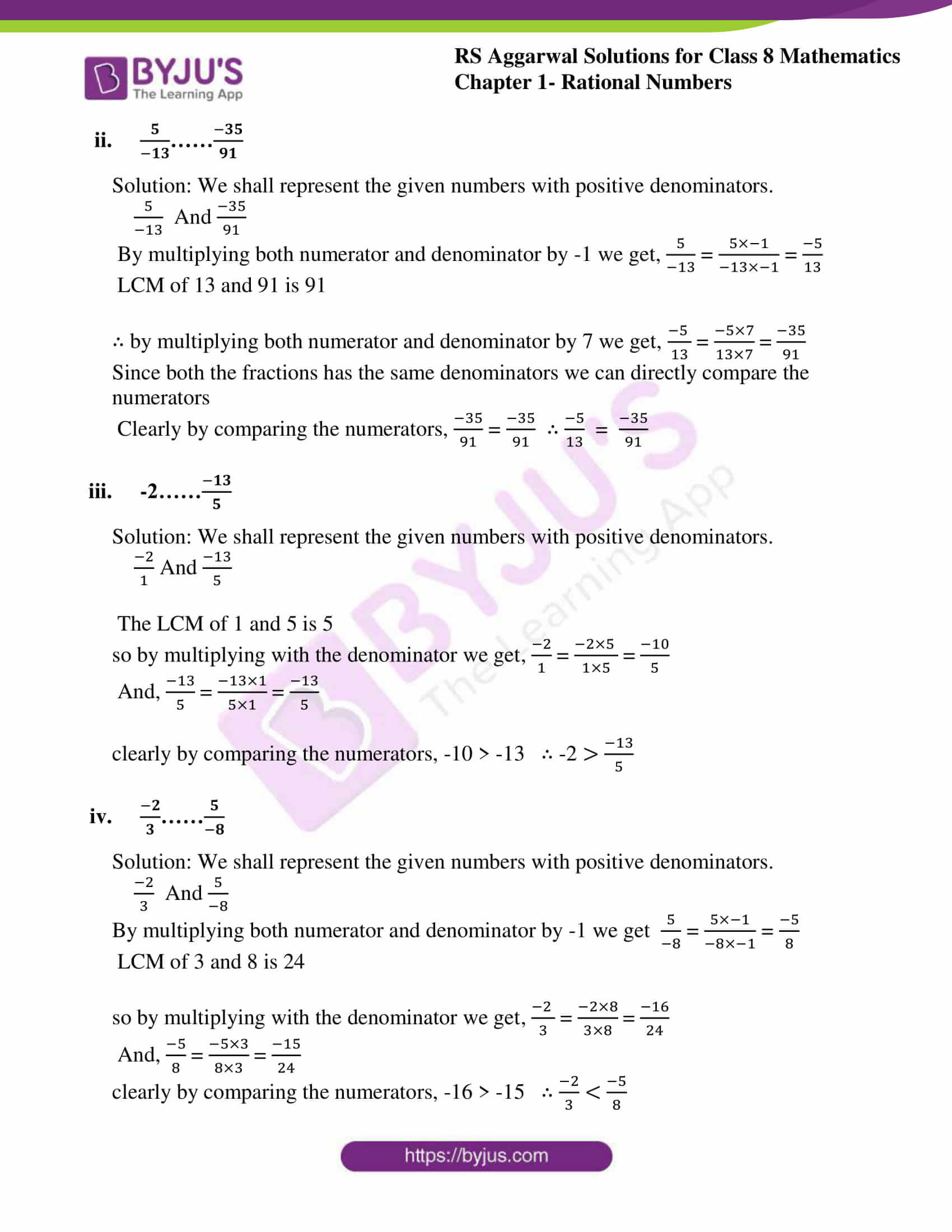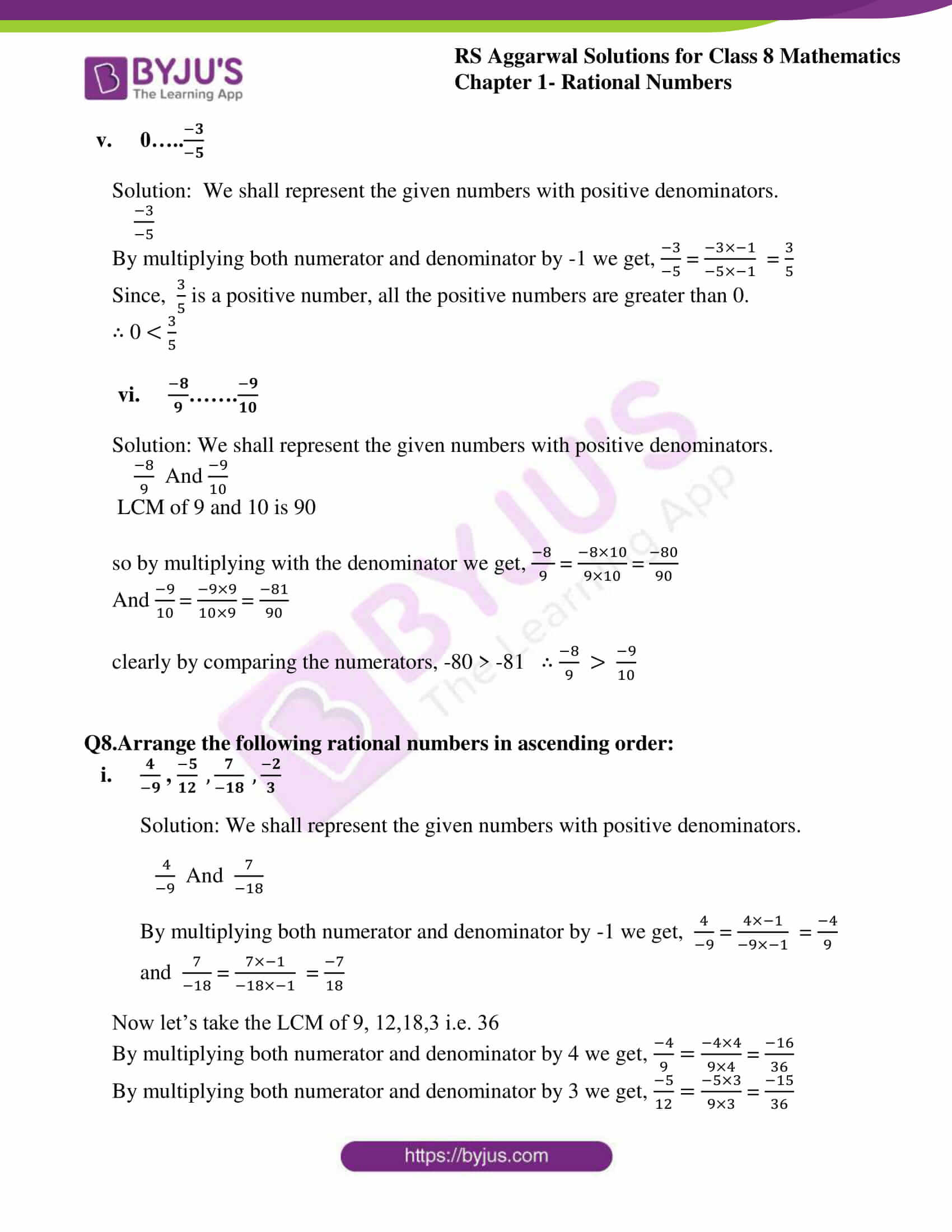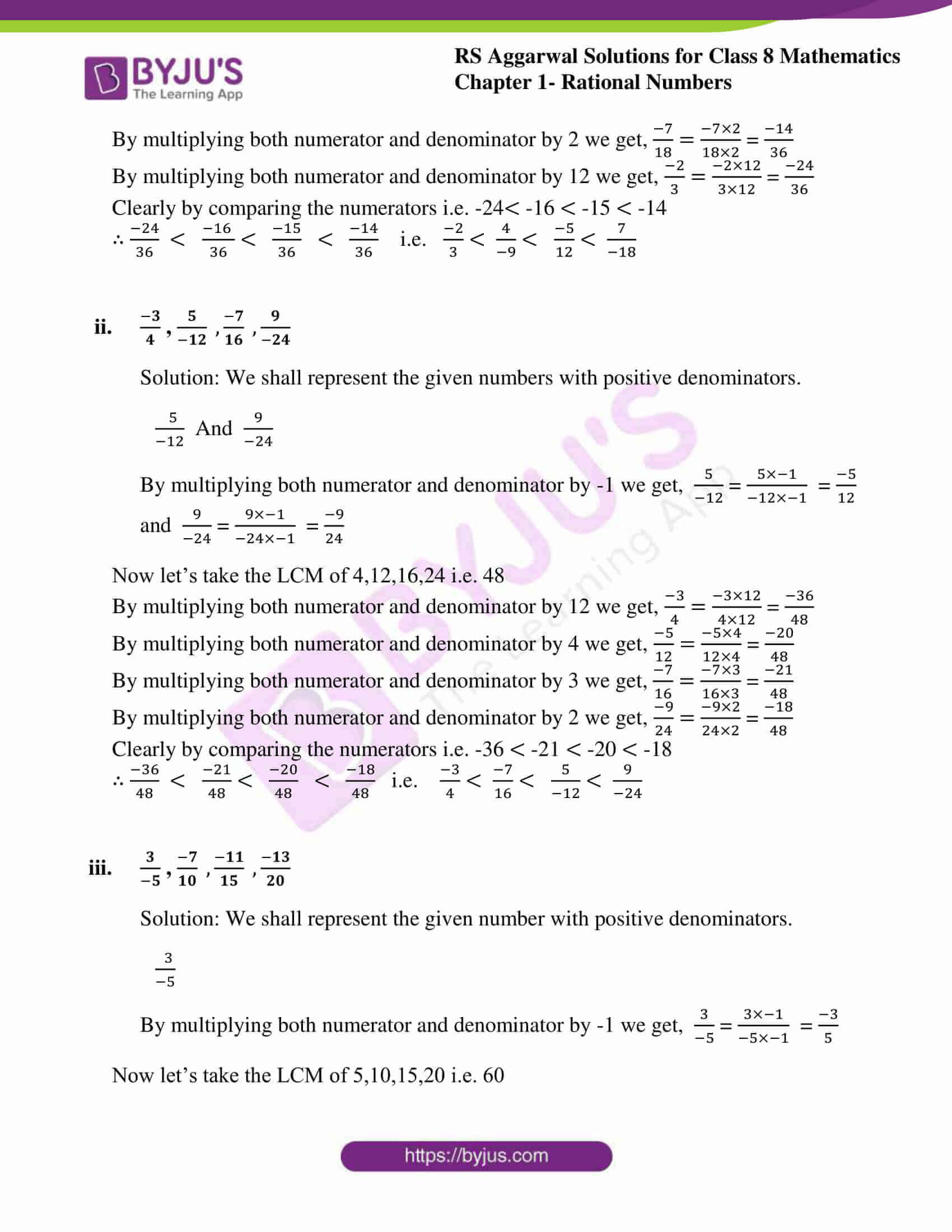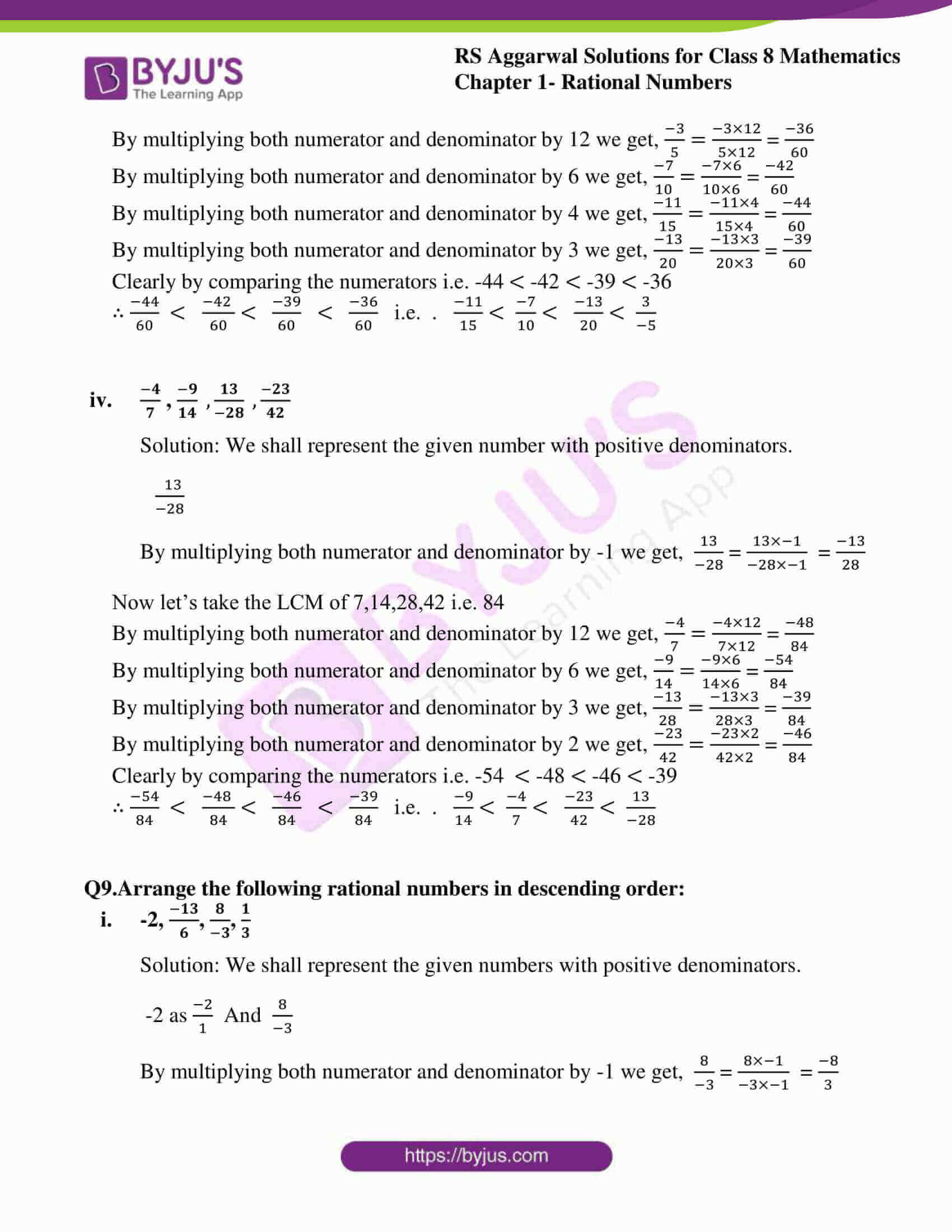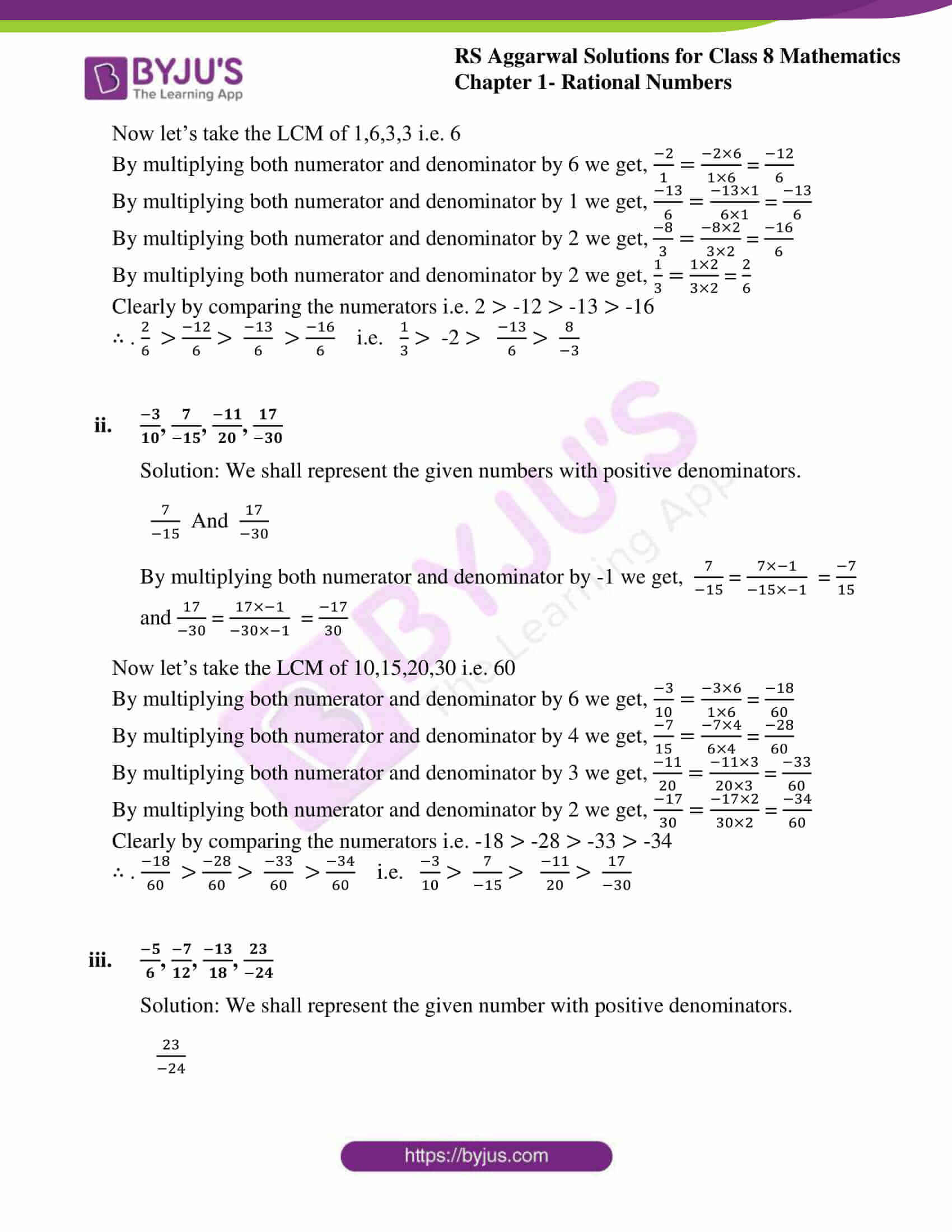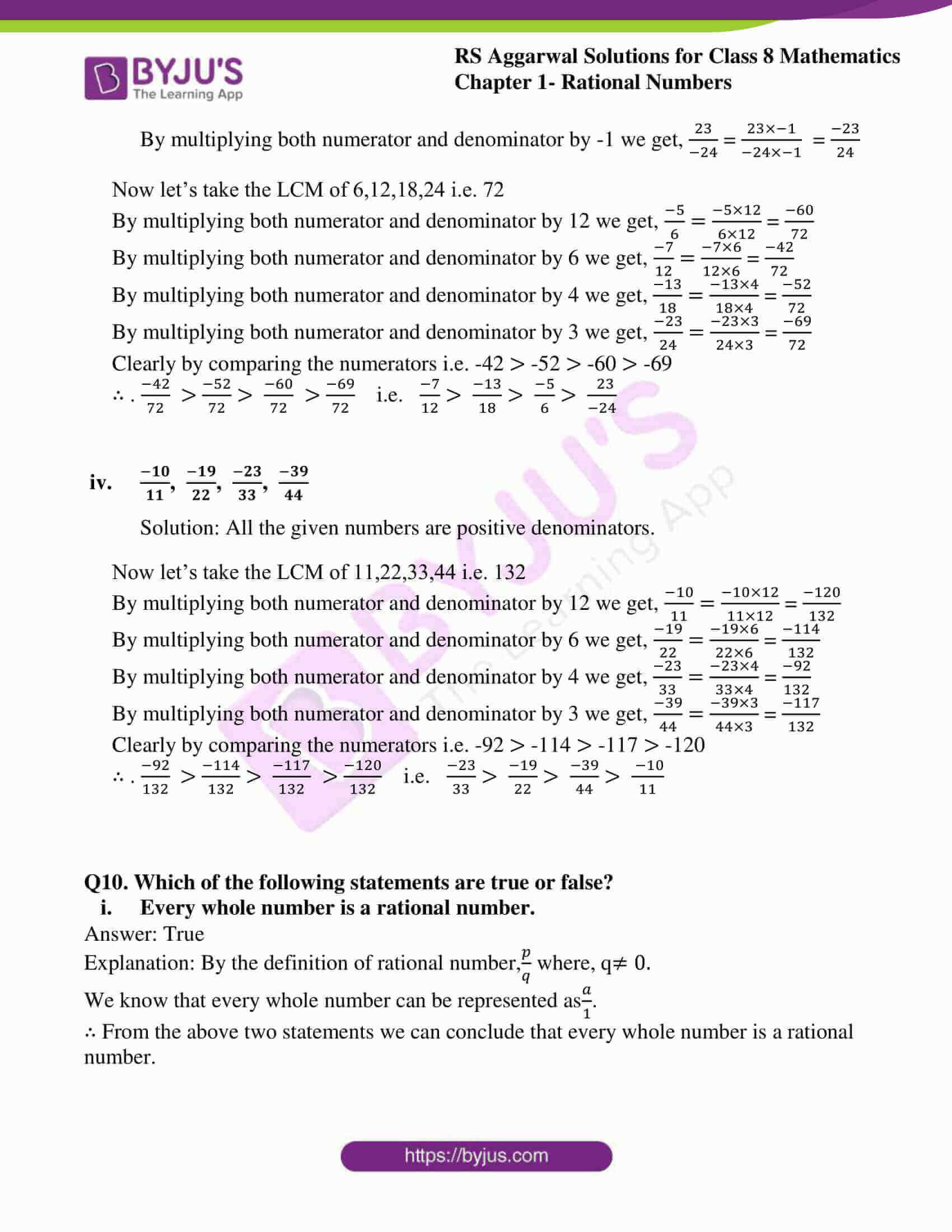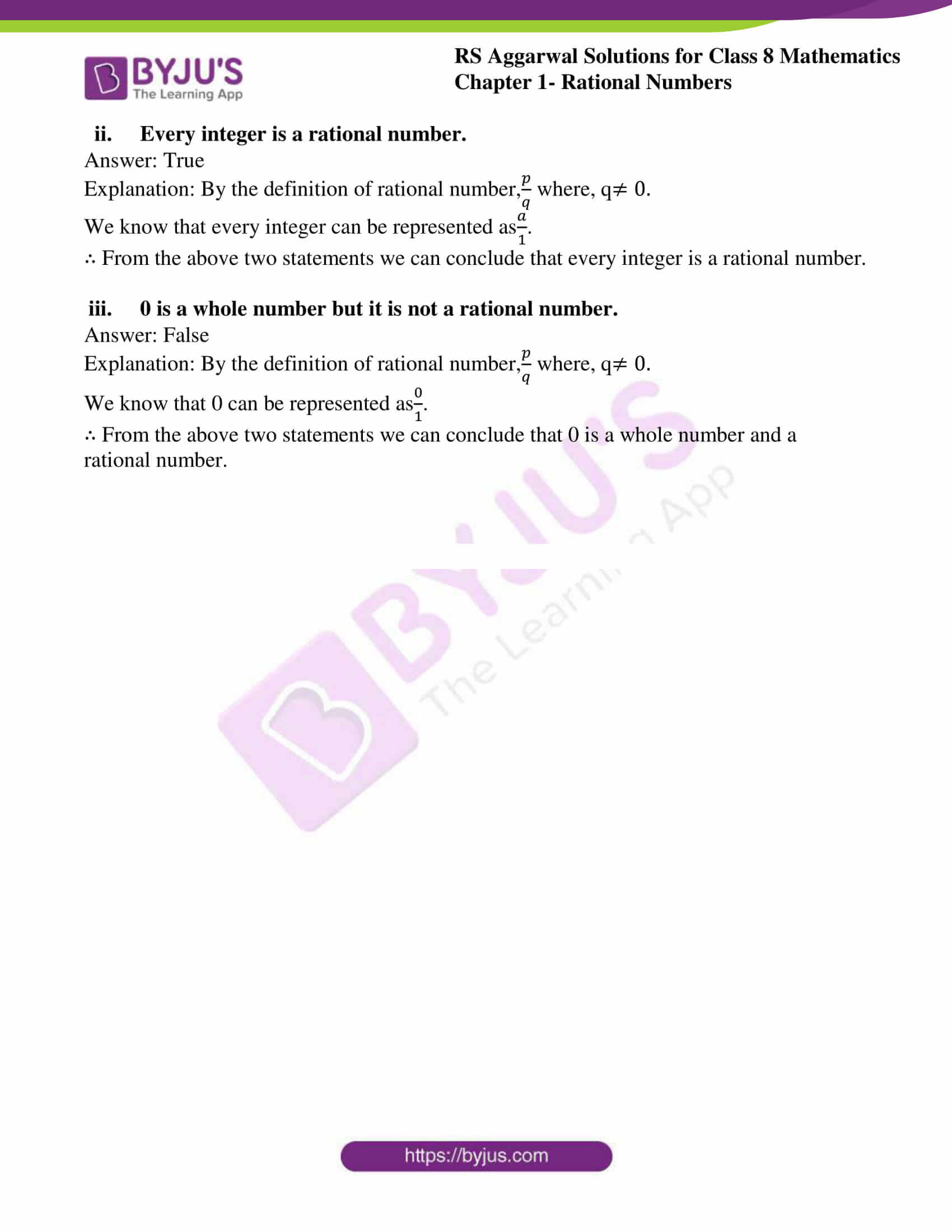### Access Answers to RS Aggarwal Solutions for Class 8 Maths Chapter 1- Rational Numbers Exercise 1A

Q1.Express -3/5 as a rational number whose denominators are: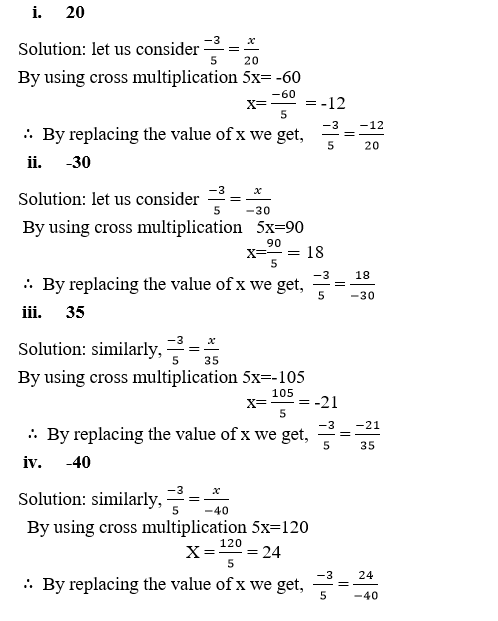Q2. Express -42/98 as a rational number whose denominator is 7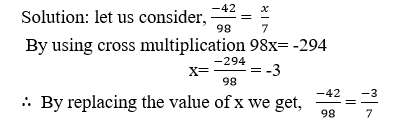Q3. Express -48/60 as a rational number whose denominator is 5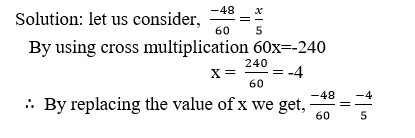Q4.express each of the following rational numbers in the standard form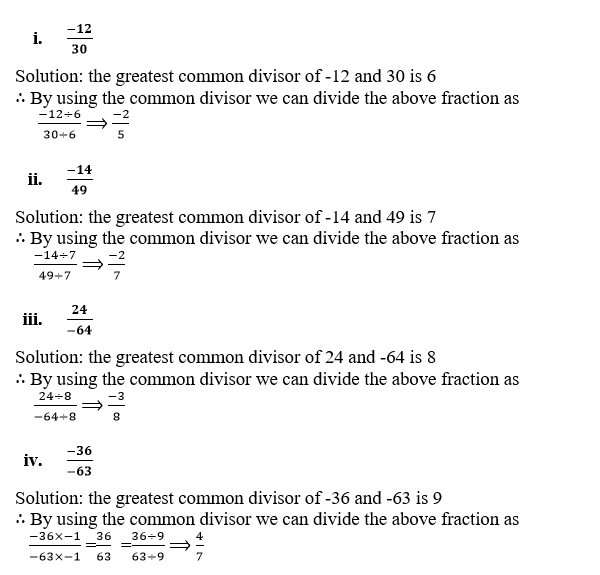Q5. Which of the two rational numbers is greater in the given pair?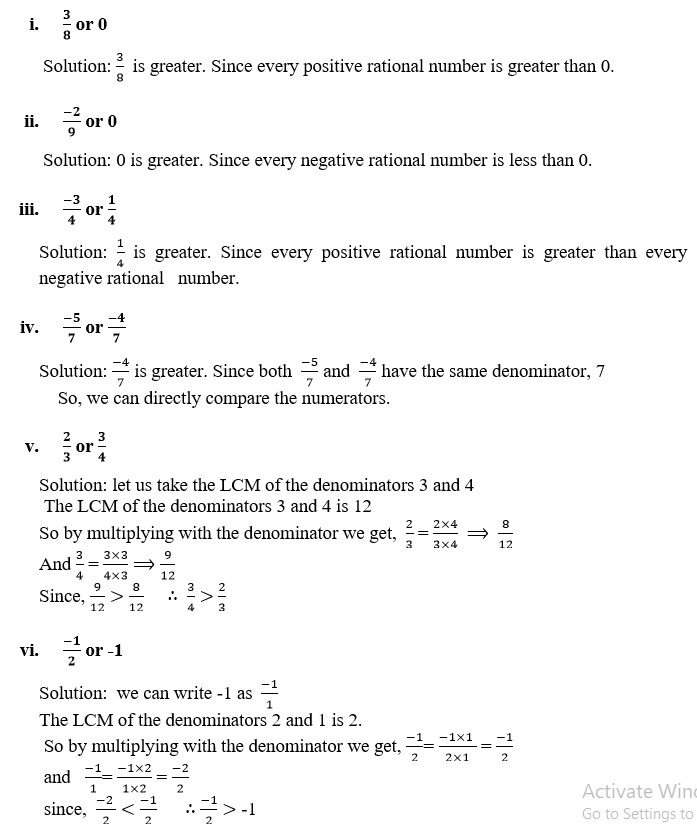Q6.which of the two rational numbers is greater in the given pair?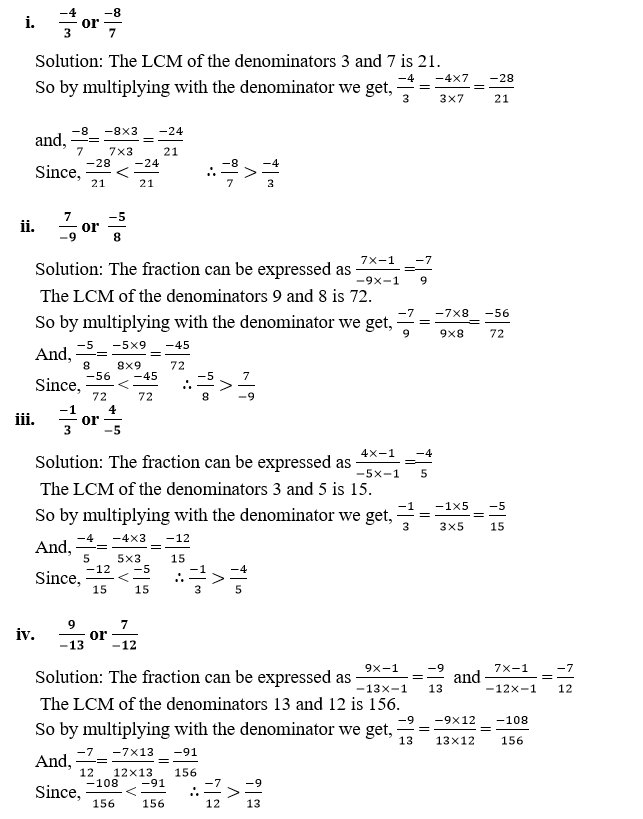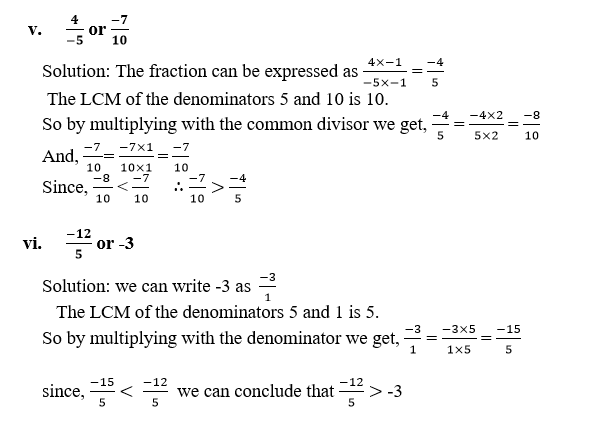Q7. Fill in the blanks with the correct symbol out of >, =, < :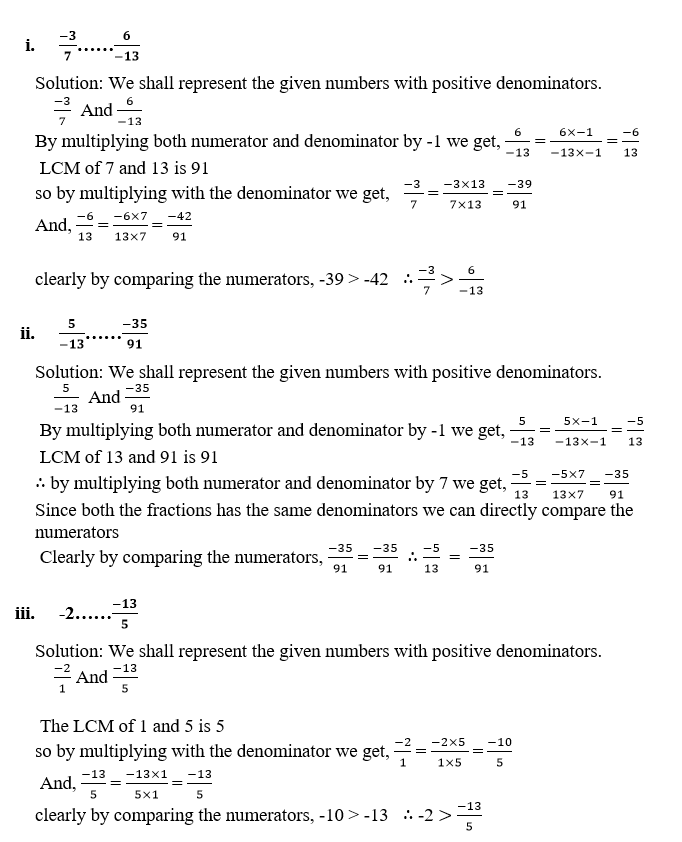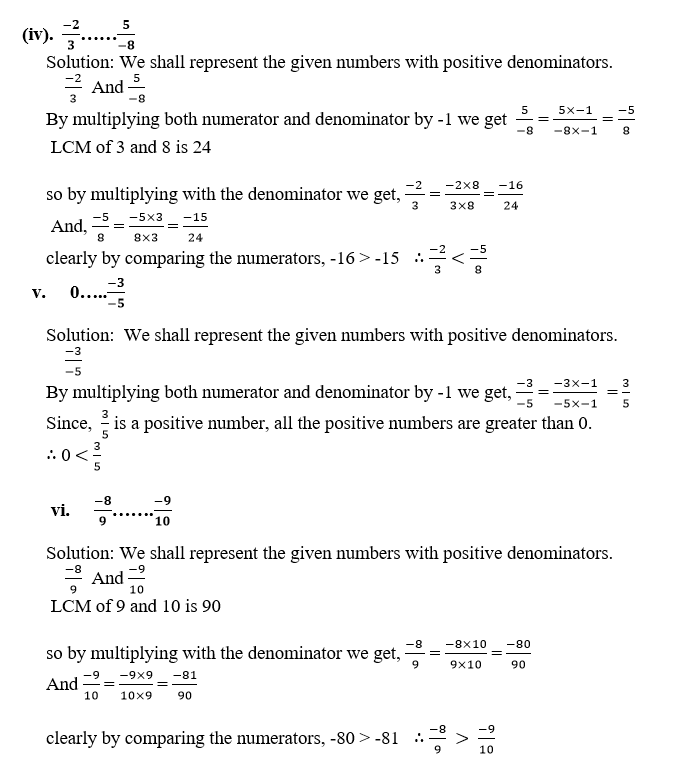Q8.Arrange the following rational numbers in ascending order: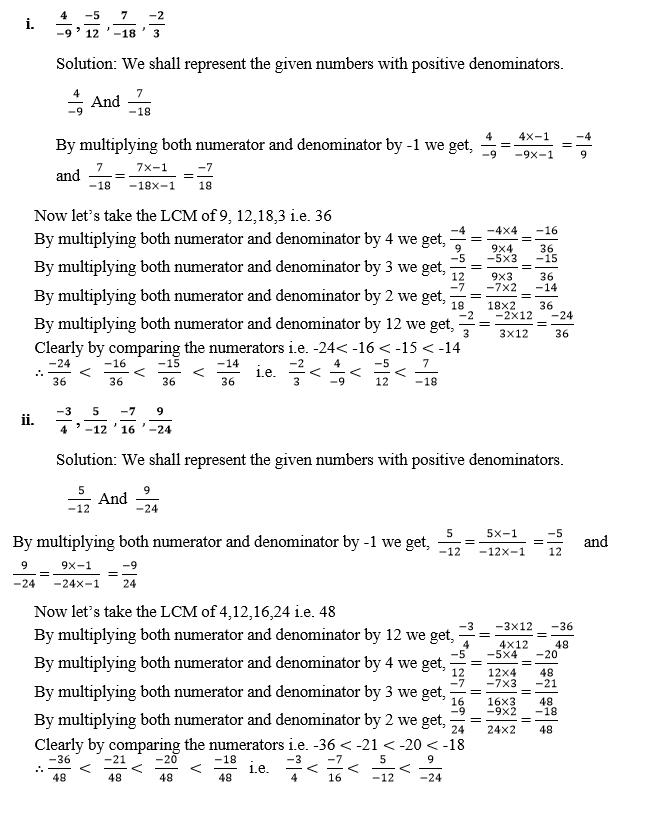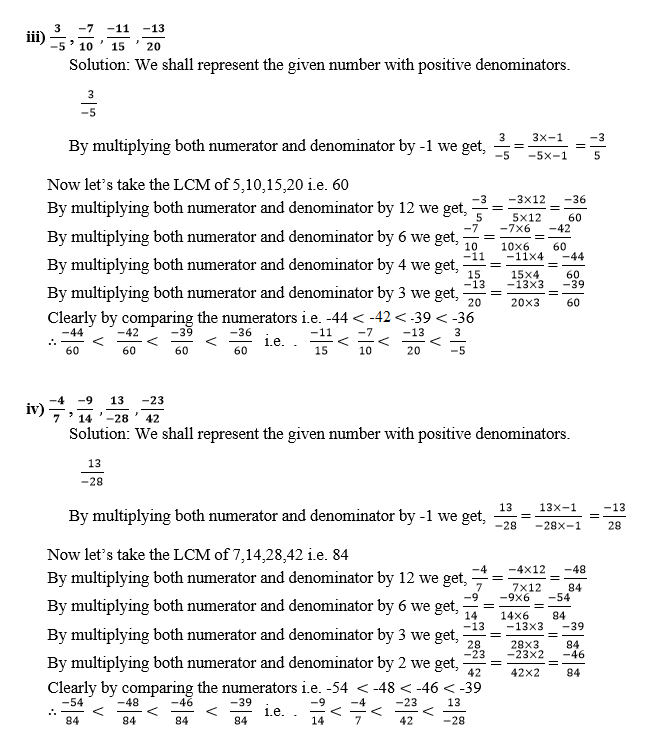Q9.Arrange the following rational numbers in descending order: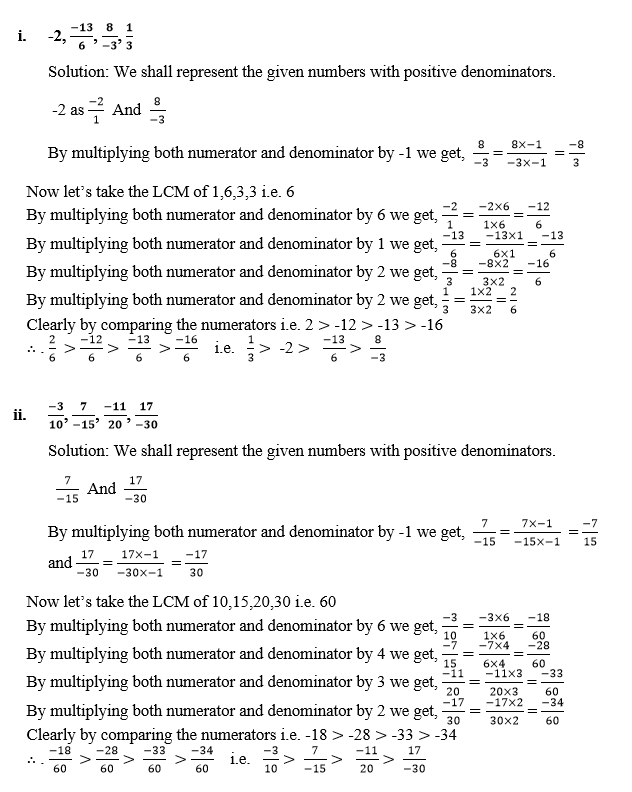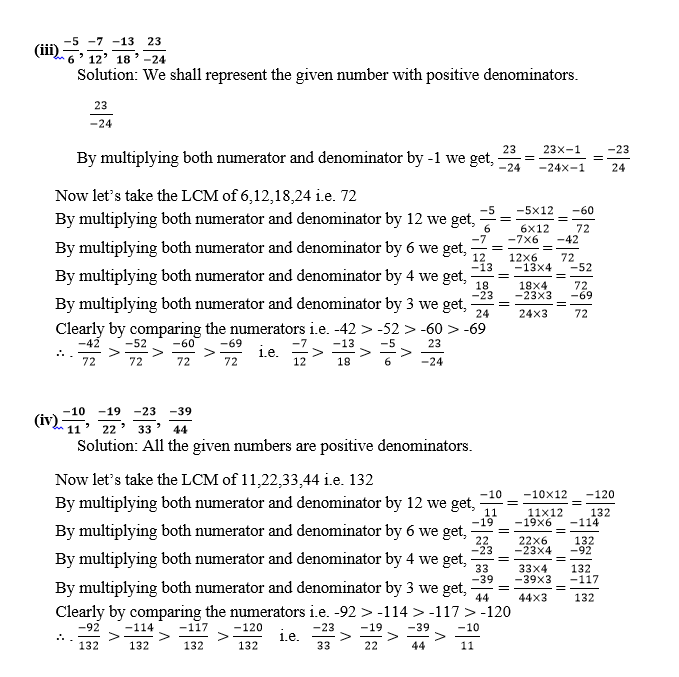Q10. Which of the following statements are true or false?

1. Every whole number is a rational number.Answer: TrueExplanation: By the definition of rational number, p/q where, q &#8800; 0.We know that every whole number can be represented as a/1.From the above two statements we can conclude that every whole number is a rational number.
2. Every integer is a rational number.Answer: TrueExplanation: By the definition of rational number, p/q where, q &#8800; 0.We know that every integer can be represented as a/1From the above two statements we can conclude that every integer is a rational number.
3. 0 is a whole number but it is not a rational number.Answer: FalseExplanation: By the definition of rational number, p/q where, q &#8800; 0.We know that 0 can be represented as 0/1.From the above two statements we can conclude that 0 is a whole number and a rational number.

### Access other Exercises of RS Aggarwal Solutions for Class 8 Maths Chapter 1- Rational Numbers

Exercise 1B Solutions 3 Questions

Exercise 1C Solutions 14 Questions

Exercise 1D Solutions 9 Questions

Exercise 1E Solutions 12 Questions

Exercise 1F Solutions 8 Questions

Exercise 1G Solutions 20 Questions

Exercise 1H Solutions 23 Questions

### RS Aggarwal Solutions for Class 8 Maths Chapter 1- Rational Numbers Exercise 1A

Exercise 1A of RS Aggarwal Class 8 Chapter 1, Rational Numbers contains the basic concepts related to Rational Numbers. This exercise mainly deals with the basic properties of Rational Numbers. Some of them include

• Equivalent Rational Numbers
• Positive and negative Rational Numbers
• Comparison of Rational Numbers

The RS Aggarwal Solutions can help the students practise diligently while learning the fundamentals, as it provides all the answers to the questions from the RS Aggarwal textbook. Practising as many times as possible helps in scoring high marks.